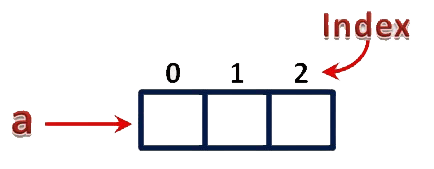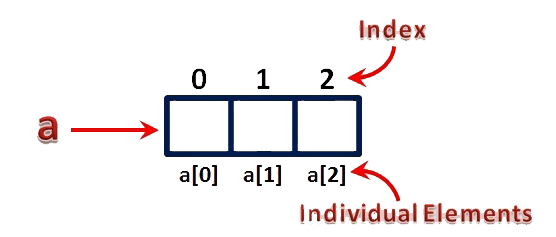The perfect place for easy learning...

×

# Arrays in C

When we work with a large number of data values we need that any number of different variables. As the number of variables increases, the complexity of the program also increases and so the programmers get confused with the variable names. There may be situations where we need to work with a large number of similar data values. To make this work easier, C programming language provides a concept called "Array".

An array is a special type of variable used to store multiple values of same data type at a time.

An array can also be defined as follows...

An array is a collection of similar data items stored in continuous memory locations with single name.

# Declaration of an Array

In C programming language, when we want to create an array we must know the datatype of values to be stored in that array and also the number of values to be stored in that array.

We use the following general syntax to create an array...

datatype arrayName [ size ] ;

Syntax for creating an array with size and initial values

datatype arrayName [ size ] = {value1, value2, ...} ;

Syntax for creating an array without size and with initial values

datatype arrayName [ ] = {value1, value2, ...} ;

In the above syntax, the datatype specifies the type of values we store in that array and size specifies the maximum number of values that can be stored in that array.

# Example Code

````int a  ;`
```

Here, the compiler allocates 6 bytes of contiguous memory locations with a single name 'a' and tells the compiler to store three different integer values (each in 2 bytes of memory) into that 6 bytes of memory. For the above declaration, the memory is organized as follows...In the above memory allocation, all the three memory locations have a common name 'a'. So accessing individual memory location is not possible directly. Hence compiler not only allocates the memory but also assigns a numerical reference value to every individual memory location of an array. This reference number is called "Index" or "subscript" or "indices". Index values for the above example are as follows...# Accessing Individual Elements of an Array

The individual elements of an array are identified using the combination of 'arrayName' and 'indexValue'. We use the following general syntax to access individual elements of an array...

arrayName [ indexValue ] ;

For the above example the individual elements can be denoted as follows...For example, if we want to assign a value to the second memory location of above array 'a', we use the following statement...

# Example Code

````a  = 100 ;`
```

The result of the above assignment statement is as follows...